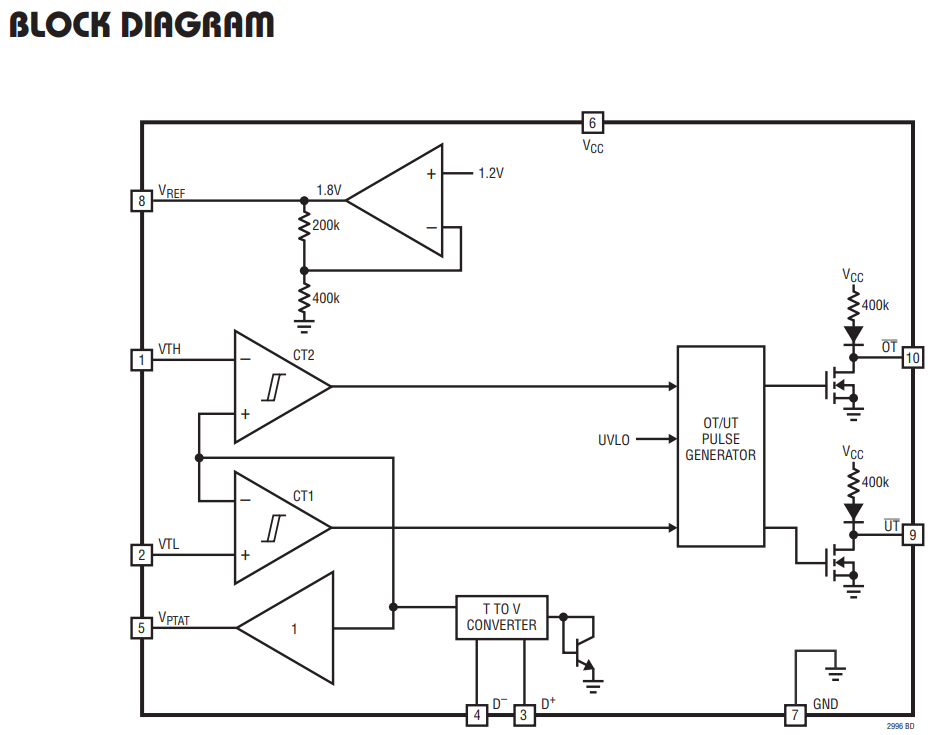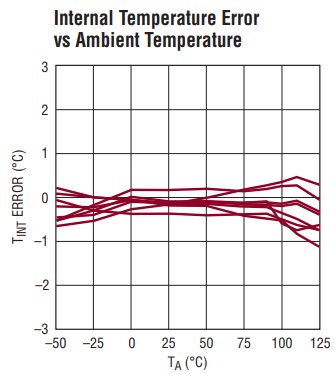# Sensors

## Introduction

Over the years, many properties of physical systems have been indentified. If they can be measured then clearly there is an instrument that performs the measurement. In many instances, such measurements can be given an electrical presence such as a voltage, a current or a resistance. When this occurs, the measuring device can become part of an IoT system.

Not all properties are of interest to an IoT system. Possibly some of them never will be. But the ability to measure a physical property by an electrical one opens up the possibility.

Wikipedia has an enormous list of sensors . These are categorised into the general types of:

• Acoustic, sound, vibration
• Automotive, transportation
• Chemical
• Electric current, electric potential, magnetic, radio
• Flow, fluid velocity
• Position, angle, displacement, distance, speed, acceleration
• Optical, light, imaging, photon
• Pressure
• Force, density, level
• Thermal, heat, temperature
• Proximity, presence
• Other

Just under, say, the category of "Proximity and presence" sensors Wikipedia lists

• Alarm sensor
• Motion detector
• Occupancy sensor
• Proximity sensor
• Passive infrared sensor
• Reed switch
• Stud finder
• Triangulation sensor
• Touch switch
• Wired glove

## Types of temperature sensor

If we drill down further into one particular type of sensor, we still see a huge variation. Temperature sensors are described by ElctronicTutorials. Firstly there are ON/OFF sensors which change from one state to the other when a temperature threshold is reached. Alternatively, a sensor may pass through a series of states (possibly continuous) as the temperature changes.

Sensors may also be of contact or non-contact types. The contact type "use conduction to measure changes in temperature," while the non-contact type "use convection and radiation to monitor changes in temperature."

Within these divisions, ElectronicTutorial further divides each one into Thermostat, Thermistor, Resistive Temperature Detectors (RTD) and Thermocouple and mentions Semiconductor Junction Sensors, Infra-red and Thermal Radiation Sensors, Medical type Thermometers, Indicators and Colour Changing Inks or Dyes Electro-mechanical, Resistive and Electronic and discusses different types within these categories. The Budding Electonic Engineer's Knowledge Space: Temperature Sensors – Types, Working & Operation goes into more detail about Semiconductor sensors

They are classified into different types like Voltage output, Current output, Digital output, Resistance output silicon and Diode temperature sensors. Modern semiconductor temperature sensors offer high accuracy and high linearity over an operating range of about 55°C to +150°C. Internal amplifiers can scale the output to convenient values, such as 10mV/°C. They are also useful in cold-junction compensation circuits for wide temperature range thermocouples.

Electronic Design: Digital Temperature Sensors gives a concise description of the principles behind Silicon temperature sensors:

Some silicon temperature sensors take advantage of the principle that when two identical transistors are operated at a constant ratio of collector current densities, the difference in their base-emitter voltage is proportional only to absolute temperature. Other sensors are based on the behavior of the base-emitter voltage, VBE, of a diode-connected transistor, which varies inversely with its temperature. The rate at which this voltage varies is a very consistent –2 mV/°C, but the absolute value of VBE varies from transistor to transistor. Compensating for this variation involves comparing ?VBE at different values of IE.

The Budding Electonic Engineer's Knowledge Space: Temperature Sensors – Types, Working & Operation goes into more detail about Silicon temperature sensors:

A silicon temperature sensor is an integrated circuit, and can therefore include extensive signal processing circuitry within the same package as the sensor. There is no need to add compensation circuits for temperature sensor Ics. Some of these are analogue circuits with either voltage or current output. Others combine analogue-sensing circuits with voltage comparators to provide alert functions. Some other sensor ICs combine analogue-sensing circuitry with digital input/output and control registers, making them an ideal solution for microprocessor-based systems.

## Properties of temperature sensors

Temperature sensors have a small range of electrical properties

• Temperature range e.g 0°C - 100°C
• Accuracy within each stated range e.g. 0°C - 100°C up to ±0.1°C
• Linearity within the range
• Interface type e.g. I2C
• Cost
• Supply voltage and current and tolerance within this e.g. "Low supply current: <420 µA (standby <0.14 µA)"
• Size
• Anticipated use e.g. automotive, HVAC, heating/cooling systems

## Some theory, and how it pans out

The following discussion is based on Chapter 7 "Sensors and Actuators" of the book INTRODUCTION TO EMBEDDED SYSTEMS A CYBER-PHYSICAL SYSTEMS APPROACH by Edward Ashford Lee and Sanjit Arunkumar Seshia, and the data sheet for a typical temperature sensor, the LTC2996 from Linear Technology (now absorbed by Analog Devices).

### Affine function

A sensor will produce values for some property, measured at some time. If the physical property is $x\left(t\right)$ at time $t$ then the sensor will report its value as $f\left(x\left(t\right)\right)$ where $f: ℝ \to ℝ$ is a function. Ideally, $f$ might be linear, but for many sensors, $f$ is an affine function

```	$f\left(x\left(t\right)\right) =ax\left(t\right) +b$
```
for some $a$ and $b$.

The LTC2996 states that it gives an output in volts for a temperature in degrees Kelvin as

```	V = T * 4/1000
```
(actually, $T$KELVIN = VPTAT / 4mV/K ) In degrees Centigrade this is
```	V = (T - 273.15) * 4/1000
```
(so that a = 4/1000 and b = -273.15*4/1000)

[Under the hood, the actual sensor chip may not behave so nicely, and Wikipedia suggests that it is better modelled by the Steinhart–Hart equation. However, after it has been processed by the LTC2996 "T to V converter" in the Block diagram it has the affine function behaviour.]### Upper and lower bounds

Sensors have upper and lower bounds in which they operate. This is not reflected in the affine formula. This would need to be modified to

```	f(x(t)) = ax(t) + b if L ≤ x(t) ≤ H
aH + b if x(t) > H
aL + b if x(t) < L,
```
where L, H ∈ ℝ, L < H, are the low and high end of the sensor range, respectively.

How the LTC2996 manages this is by several means

• By setting appropriate voltages on the VTL and VTH pins, the minimum and maximum values respectively can be set
• When the temperature exceeds the value set by VTH, the sensor triggers an alert function: the OT pin is pulled to GND and similarly for UT when the temperature falls below the value set by VTL
• Monitoring the voltages on OT and UT will show if the temperature readings are out of range

### Refreshing measurements

The value of the temperature is not the instantaneous value of the temperature. Instead, sample test currents are sent through the transistor and the voltage measured over a period of 3.5ms and the temperature reported is refreshed every 3.5ms based on these values. The temperature reported is effectively by intervals: at any time it is the average of the temperature over the previous 3.5ms period.

In most circumstances this will not matter, unless timing for a more precise period is required. This is an artefact of the way the temperature is measured and is not related to quantisation effects caused by digitising an analogue signal: this is discussed later. Note that this digitisation is of the time axis, not of the value axis as occurs in digitising the analogue signal.

However, there is another factor which could be more important. The sensor is able to use an onboard or external NPN transistor. An external transistor can allow measurements to be taken some distance away from the sensor chip itself. That transistor may be shielded or insulated. In that case, temperature changes have to penetrate through the shielding. The page Thermocouple Response Time by Omega gives graphs of the time delays introduced by shielding a thermocouple, showing that if the sheath diameter is, say, over 0.032 inches, then the delay will be about 2 seconds.

### Noise

Noise is an inaccuracy in what we are trying to measure. It will be some function n(t) giving an incorrect value of f(x(t)):

```	f(x(t)) = x(t) + n(t)
```
Various charts are given for the LTC2996, such asNoise can be reduced by averaging the temperature over a period. To reduce the noise of the LTC2996 to, say, 0.01°C RMS, an averaging period of one second is recommended. This may or may not have implications for an IoT system.

### Digital quantization

The LTC2996 is an analogue sensor. For use in an IoT system the temperature will need to be converted to a digital signal. This then introduces the issue that arises when digitising any analogue signal, and that is the quantisation effect.

Suppose the signal is digitised using a 3-bit value. That would divide the range into only 8 segments. That is, if the range of analogue measurements was 0-80°C, then digitisation would place a measurement into one of 10°C blocks. More realistically, 16 bits will be used, which would give a block size of 0.00122°C. The page digital sensors lists many digital sensors including the number of bits and corresponding resolution.

## Messaging protocols

A sensor such as a digital temperature sensor will produce electronic behaviour which has to be managed by whatever it is connected to, such as a microcontroller. Each sensor will require a different management mechanism. There are a variety of protocols which abstract such behaviour. See Communication Buses and Protocols for Sensor Networks

### I²C